# Graph Theory - Examples

#### Graph Theory

97 Lectures 7 hours

#### Graph Theory Algorithms

32 Lectures 6.5 hours

In this chapter, we will cover a few standard examples to demonstrate the concepts we already discussed in the earlier chapters.

## Example 1

Find the number of spanning trees in the following graph.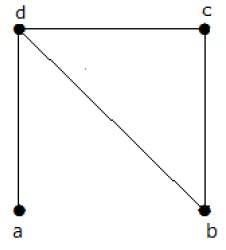### Solution

The number of spanning trees obtained from the above graph is 3. They are as follows −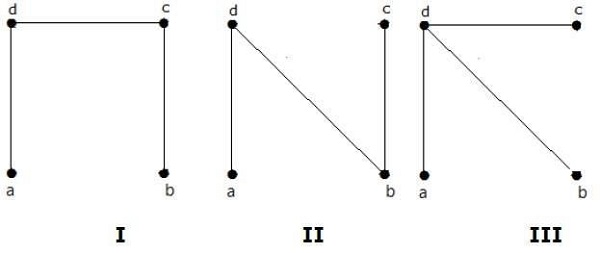These three are the spanning trees for the given graphs. Here the graphs I and II are isomorphic to each other. Clearly, the number of non-isomorphic spanning trees is two.

## Example 2

How many simple non-isomorphic graphs are possible with 3 vertices?

### Solution

There are 4 non-isomorphic graphs possible with 3 vertices. They are shown below.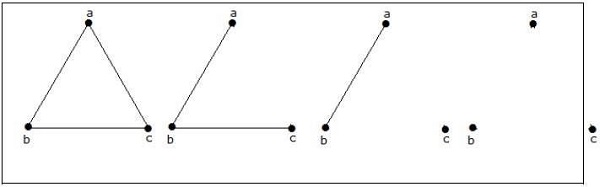## Example 3

Let ‘G’ be a connected planar graph with 20 vertices and the degree of each vertex is 3. Find the number of regions in the graph.

### Solution

By the sum of degrees theorem,

20 Σ i=1 deg(Vi) = 2|E|

20(3) = 2|E|

|E| = 30

By Euler’s formula,

|V| + |R| = |E| + 2

20+ |R| = 30 + 2

|R| = 12

Hence, the number of regions is 12.

## Example 4

What is the chromatic number of complete graph Kn?

### Solution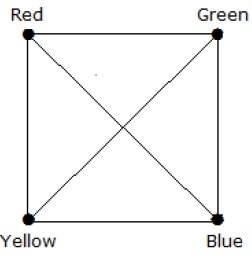In a complete graph, each vertex is adjacent to is remaining (n–1) vertices. Hence, each vertex requires a new color. Hence the chromatic number Kn = n.

## Example 5

What is the matching number for the following graph?

### Solution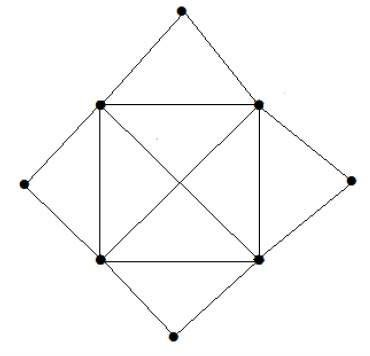Number of vertices = 9

We can match only 8 vertices.

Matching number is 4.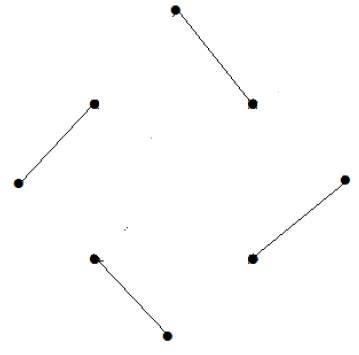## Example 6

What is the line covering number of for the following graph?

### Solution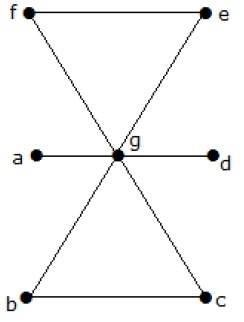Number of vertices = |V| = n = 7

Line covering number = (α1) ≥ [n/2] = 3

α1 ≥ 3

By using 3 edges, we can cover all the vertices.

Hence, the line covering number is 3.